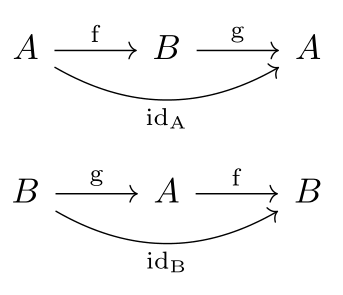Show Question
Math and science::Algebra::Aluffi

# Injectivity and surjectivity

### Injective

A function $$f : A \to B$$ is injective iff

$(\forall a' \in A)(\forall a'' \in A) \quad a' \neq a'' \implies f(a') \neq f(a'')$

Injections are often denoted like $$A \hookrightarrow B$$.

### Surjective

A function $$f : A \to B$$ is surjective iff

$(\forall b \in B) (\exists a \in A) \quad b = f(a)$

Surjections are often denoted like $$A \twoheadrightarrow B$$.

### Bijective

A function $$f$$ that is both injective and surjective is said to be bijective. A bijective function is called a bijection or a one-to-one correspondence or an isomorphism of sets; it is is often denoted like $$f : A \xrightarrow{\sim} B$$.

If there is a bijection between sets $$A$$ and $$B$$, then each element $$a$$ of $$A$$ can be matched with exactly one element $$b$$ of $$B$$. $$A$$ and $$B$$ are said to be isomorphic. The statement that $$A$$ and $$B$$ have such a relationship is often denoted as $$A \cong B$$.

### From the perspective of inverses

If $$f : A \to B$$ is a bijection, it can be 'flipped' to define a function $$g : B \to A$$. This is a flipping of $$\Gamma_f$$, the graph of $$f$$, along it's diagonal. $$f$$ being a bijection insures that such a flipping action produces a valid function. The function $$g$$ has two interesting properties:

$g \circ f = \operatorname{id_A}$
$f \circ g = \operatorname{id_B}$

These properties correspond to the statement that the following two diagrams compute:#### Bijections have inverses

The first identity above states that $$g$$ is a left-inverse of $$f$$. The second identity states that $$g$$ is a right-inverse of $$f$$. Combined we say that $$g$$ is the inverse of $$f$$, and denote it as $$f^{-1}$$. Thus, bijections have inverses.

#### If a function has an inverse, is it a bijection?

This is true. We can, in fact, break this statement into two pieces.

Let $$A$$ and $$B$$ be sets, with $$A \neq \emptyset$$, and let $$f : A \to B$$ be a function. Then the following bi-implications hold:

1. $$f$$ has a left-inverse if and only if it is injective.
2. $$f$$ has a right-inverse if and only if it is surjective.

Can you think of the proof of these two bi-implications?

Futhermore, if $$f$$ has a left-inverse $$g_l$$ and right-inverse $$g_r$$, then these inverses must be the same function! This can be shown by considering:

$g_l = g_l \operatorname{id_B} = g_l (f g_r ) = (g_l f) g_r = \operatorname{id_A} g_r = g_r$

### Essense of a injectivity and surjectivity

The proposition above hints at something fundamental at play. By the proposition above injectivity and surjectivity can be phrased in terms of how functions are organized, not in terms of the specific elements of the sets. As Aluffi mentions, this is a more 'mature' point of view from which to perceive functions.

### Injective $$\iff$$ has a left-inverse. Proof.

Let $$f : A \to B$$ be a function. $$f$$ has a left-inverse if and only if it is injective.

Proof. [Note: I think this uses an the axiom of choice for the second part.]

($$\impliedby$$). Assume $$f$$ has a left-inverse. This means that there is a function $$g : B \to A$$ such that $$g \circ f = \operatorname{id_A}$$. Let $$a_1, a_2 \in A$$ be two distinct elements of $$A$$ ($$a_1 \neq a_2$$). We know that $$\operatorname{id_A}(a_1) \neq \operatorname{id_A}(a_2)$$, so it must be that $$g \circ f(a_1) \neq g \circ f(a_2)$$. This can only be so if $$f(a_1) \neq f(a_2)$$. So $$f$$ is injective.

($$\implies$$). Assume that $$f$$ is injective. We must find a function that meets the criteria of being a left-inverse of $$f$$. Choose the function $$g : B \to A$$ defined by:

$g(b) = \begin{cases} a & \text{if } f(a) = b \text{ for some } a \in A \\ \text{any element of A} & \text{if } b \notin \operatorname{im} f \end{cases}$

You can check that this defines a function and acts as a left-inverse for $$f$$.

### Surjective $$\iff$$ has a right-inverse. Proof.

This is a similar proof to the one above. Maybe I'll add the details one day.